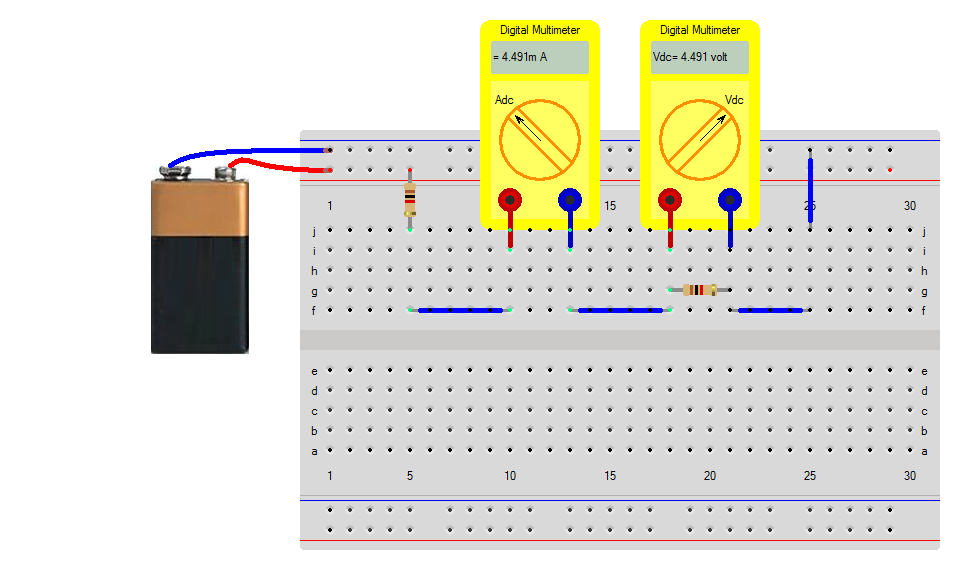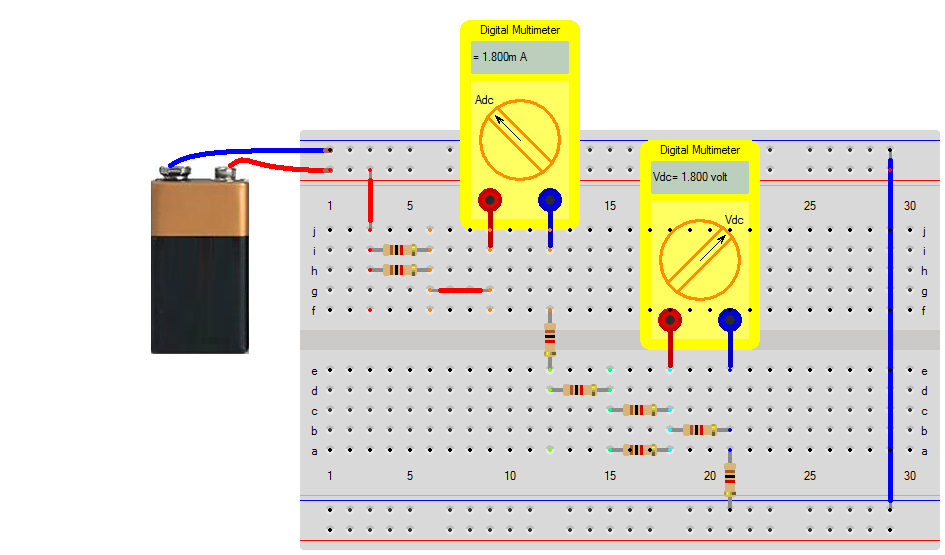• Introduction
• Power Electronics
• SMPS
• DC Drives
• AC Drives
• Drives
• Green Energy

Series and parallel connection of resistors

The series and parallel connection of resistors is elaborated in this tutorial. We are going to make a simple resistive circuits and measure voltage and current. The purpose of this tutorial is to get acquainted with the basic editing modes when creating a breadboard simulation and to get some practical experience when using the breadboard.

Series connection of 1kΩ

The simulation will contain 2 resistors of 1kΩ in series connected to a 9v battery. The total current through the resistors will be $i=\frac{9}{2 \cdot 1k} = 4.5 mA$ and the voltage over a single resistor will be $v= \frac{1k\Omega}{1k\Omega + 1k\Omega} \cdot 9v = 4.5v$ or alternatively $v= 1k \cdot i$Note that he battery is not exactly 9v. There is some internal resistance and since the battery voltage is also degrading after some use, the battery-voltage is always slightly lower than 9v.

Also there is some wire resistance. Each wire can have a resistance up to 1Ω

But the largest error comes from your Digital Multimeter Meter when measuring voltage and current. Non-certified multimeters can have an error in the range of 5% to 10%.

You can give an estimation of the internal resistance of the battery as being$R_{internal}^{Battery} = \frac{9-2k \cdot i}{i} = 4\Omega$ when using $$i=4.491 mA$$ Seems like the wires have an resistance of 1Ω each.

Unequal resistor values

Now we are going to take other reistor values. Select new resistors from the Components/Library/Breadboard

The simulation will contain 2 resistors of 2k7Ω and 4k7Ω in series connected to a 9v battery. The total current through the resistors will be $i=\frac{9}{2k7\Omega + 4k7\Omega} = 1.2mA$ and the voltage over a 4k7 resistor will be $v= \frac{4k7\Omega}{2k7\Omega + 4k7\Omega} \cdot 9v = 5.7v$ or alternatively $v= 4k7\Omega \cdot 1.2mA = 5.7 volt$Parallel connection of 1kΩ

Placing two 1kω resistors in parallel creates 500Ω resistors. The voltage division is equal compared to the circuit with two 1kω resistors in series, but the current now measures $i= \frac{9v}{500 + 500} = 9 mA$Parallel and series connection of 1kΩ

The total resistance of a combination of parallel and series connections of 1kΩ resistors is calculated as $\frac{1k \cdot 1k}{1k+1k} + 1k + 1k + \frac{1k \cdot 1k}{1k+1k} + 1k +1k = 500 + 2k + 500 +2k = 5k$ The current will be $i= \frac{9}{5k} = 1.8 mA$© 2018 CASPOC, All rights reserved. Home   |   Terms and Conditions   |   Legal   |   Export Compliance   |   Privacy Policy   |   Site Map